# Difference between revisions of "11-1100/Homework Assignment 5"

Jump to: navigation, search

### The Final Exam

The Final Exam will take place on Friday December 9, 10-1 at Bahen 6183. You may expect very approximately one third of the exam to be about class-proven theorems, one third to be repeats of HW problems and/or exam problems from this year or last, and one third to be fresh exercises. You will have to solve about 5 out of about 6 problems. It is likely that the overall shape of the exam will be similar to last year's final exam, which can be found at 10-1100/Final Exam.

When I was a student, before exams I usually made sure that I absolutely understand all class material and I worried less about the exercises, on the assumption that class was about the most important knowledge and that if I really understood all that was done in class, the exercises would follow relatively easily. That was my strategy; it worked well for me, but what works for you is not for me to tell.

### Last Week's Schedule

Warning. This schedule is subject to changes. Recheck this web site the day before any activity.

 Tuesday December 6 10-12 Last Class Wednesday December 7 12-2 Dror's office hours, Bahen 6178. 2PM HW5 "early bird" due date. If you submit HW5 by this time, it will be marked by noon of the following day. Thursday December 8 10:30-12:30 Dror's office hours, Bahen 6178. Noon HW5 is due in Dror's office, to be graded after the final. Also, at this time "early bird" marked HW5 can be collected at Dror's office. 3-5 Stephen Morgan's office hours, at Huron 1028. Friday December 9 10-1 The Final Exam.

### Solve the following questions

Problem 1. Let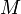$M$ be a module over a PID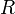$R$. Assume that$M$ is isomorphic to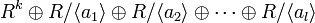$R^k\oplus R/\langle a_1\rangle\oplus R/\langle a_2\rangle\oplus\cdots\oplus R/\langle a_l\rangle$, with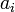$a_i$ non-zero non-units and with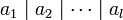$a_1\mid a_2\mid\cdots\mid a_l$. Assume also that$M$ is isomorphic to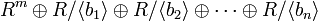$R^m\oplus R/\langle b_1\rangle\oplus R/\langle b_2\rangle\oplus\cdots\oplus R/\langle b_n\rangle$, with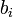$b_i$ non-zero non-units and with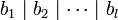$b_1\mid b_2\mid\cdots\mid b_l$. Prove that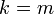$k=m$, that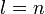$l=n$, and that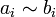$a_i\sim b_i$ for each$i$.

Problem 2. Let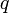$q$ and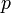$p$ be primes in a PID$R$ such that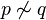$p\not\sim q$, let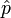$\hat{p}$ denote the operation of "multiplication by$p$", acting on any$R$-module$M$, and let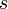$s$ and$t$ be positive integers.

1. For each of the$R$-modules$R$,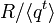$R/\langle q^t\rangle$, and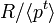$R/\langle p^t\rangle$, determine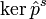$\ker\hat{p}^s$ and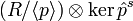$(R/\langle p\rangle)\otimes\ker\hat{p}^s$.
2. Explain why this approach for proving the uniqueness in the structure theorem for finitely generated modules fails.

Problem 3. (comprehensive exam, 2009) Find the tensor product of the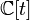${\mathbb C}[t]$ modules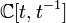${\mathbb C}[t,t^{-1}]$ ("Laurent polynomials in$t$") and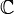${\mathbb C}$ (here$t$ acts on${\mathbb C}$ as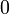$0$).

Problem 4. (from Selick) Show that if$R$ is a PID and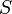$S$ is a multiplicative subset of$R$ then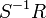$S^{-1}R$ is also a PID.

Definition. The "rank" of a module$M$ over a (commutative) domain$R$ is the maximal number of$R$-linearly-independent elements of$M$. (Linear dependence and independence is defined as in vector spaces).

Definition. An element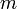$m$ of a module$M$ over a commutative domain$R$ is called a "torsion element" if there is a non-zero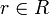$r\in R$ such that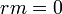$rm=0$. Let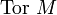$\mbox{Tor }M$ denote the set of all torsion elements of$M$. (Check that$\mbox{Tor }M$ is always a submodule of$M$, but don't bother writing this up). A module$M$ is called a "torsion module" if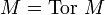$M=\mbox{Tor }M$.

Problem 5. (Dummit and Foote, page 468) Let$M$ be a module over a commutative domain$R$.

1. Suppose that$M$ has rank$n$ and that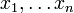$x_1,\ldots x_n$ is a maximal set of linearly independent elements of$M$. Show that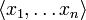$\langle x_1,\ldots x_n\rangle$ is isomorphic to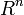$R^n$ and that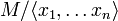$M/\langle x_1,\ldots x_n\rangle$ is a torsion module.
2. Conversely show that if$M$ contains a submodule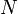$N$ which is isomorphic to$R^n$ for some$n$, and so that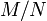$M/N$ is torsion, then the rank of$M$ is$n$.

Problem 6. (see also Dummit and Foote, page 469) Show that the ideal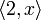$\langle 2,x\rangle$ in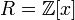$R={\mathbb Z}[x]$, regarded as a module over$R$, is finitely generated but cannot be written in the form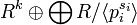$R^k\oplus\bigoplus R/\langle p_i^{s_i}\rangle$.